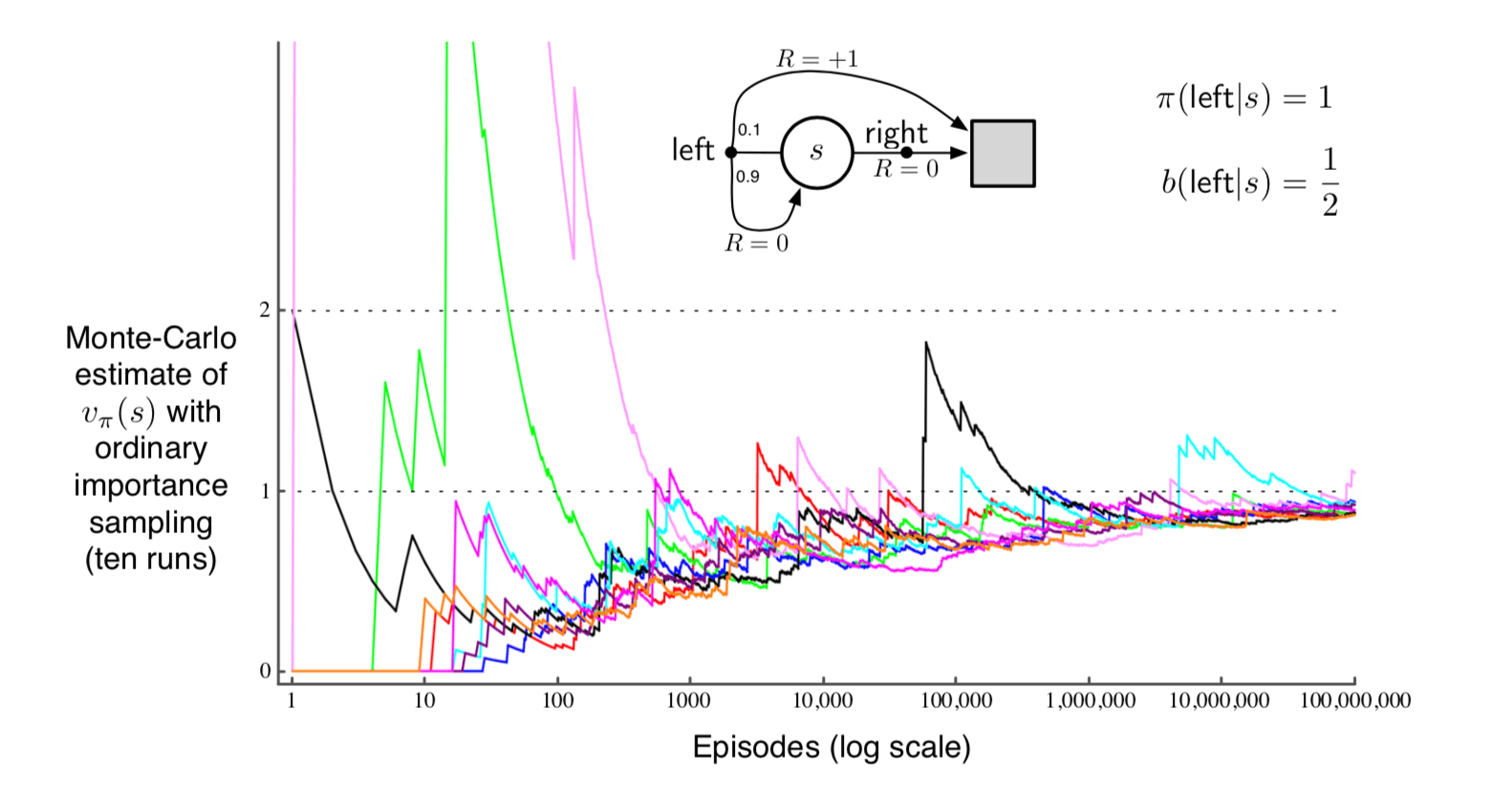# 第5章 蒙特卡洛方法¶

## 5.1 蒙特卡洛预测¶

$$Returns(s) \leftarrow$$ 一个空的列表，对所有 $$s\in\mathcal{S}$$

$$G \leftarrow 0$$

$$G \leftarrow \gamma{G} + R_{t+1}$$

$$G$$ 添加到列表 $$Returns(s)$$

$$V(s) \leftarrow average(Returns(s))$$

21点可以看成一个回合式的有限马尔可夫过程。每次游戏都是一个回合。赢、输、draw的奖励分别为1、-1、0。 游戏过程中的任意动作奖励都为0，且我们不算折扣（$$\gamma = 1$$）；因此这些结束状态的奖励即是回报。 玩家的动作只有要牌或者停止要牌两种。游戏的状态取决于玩家是什么牌以及庄家手上的明牌。 我们假设牌数是无限的，这样我们记牌就没有优势了。 如果玩家将A当成11点来算的话（不能爆掉），我们称它 可用 。 开始时我们把A当成11，因为如果当成1的话，那么开始时的牌肯定是小于等于11点的，这样我们没有更多的选择，肯定是会要牌的。 所以，玩家做出的决定依赖于三个变量：当前的牌的点数和（12-21）、庄家的明牌的点数（A-10）以及玩家是否有可用的A。 这样的话，总共有200个不同的状态。## 5.3 蒙特卡洛控制¶

$\pi_0 \overset{E}{\rightarrow} q_{\pi_0} \overset{I}{\rightarrow} \pi_1 \overset{E}{\rightarrow} q_{\pi_1} \overset{I}{\rightarrow} \pi_2 \overset{E}{\rightarrow} \cdots \overset{I}{\rightarrow} \pi_{*} \overset{E}{\rightarrow} q_{*}$

(1)$\pi(s) \dot{=} arg \space \underset{a}{max} \space q(s,a)$

\begin{split}\begin{aligned} q_{\pi_{k}}\left(s, \pi_{k+1}(s)\right) &=q_{\pi_{k}}(s, \arg \max _{a} q_{\pi_{k}}(s, a)) \\ &=\max _{a} q_{\pi_{k}}(s, a) \\ & \geq q_{\pi_{k}}\left(s, \pi_{k}(s)\right) \\ & \geq v_{\pi_{k}}(s) \end{aligned}\end{split}

$$S_0, A_0$$ 开始，遵循策略 $$\pi$$ ，生成一个回合：$$S_0, A_0, R_1, \dots , S_{T-1}, A_{T-1}, R_T$$

$$G \leftarrow 0$$

$$G \leftarrow \gamma G + R_{t+1}$$

$$G$$ 添加到 $$Returns(S_t, A_t)$$

$$Q(S_t, A_t) \leftarrow average(Returns(S_t, A_t))$$

$$\pi(S_t) \leftarrow \mathop{argmax} \limits_{a} Q(S_t, a)$$## 5.4 非探索开端的蒙特卡洛控制¶

$$\pi \leftarrow$$ 任意 $$\epsilon-soft$$ 策略

$$G \leftarrow 0$$

$$G \leftarrow \gamma G + R_{t+1}$$

$$G$$ 添加到 $$Returns(S_t, A_t)$$

$$Q(S_t, A_t) \leftarrow average(Returns(S_t, A_t))$$

$$A^* \leftarrow \mathop{argmax} \limits_{a} Q(S_t, a)$$ （随意打破关系）

$\begin{split}\pi\left(a \mid S_{t}\right) \leftarrow\left\{\begin{array}{ll} 1-\varepsilon+\varepsilon /\left|\mathcal{A}\left(S_{t}\right)\right| & \text { if } a=A^{*} \\ \varepsilon /\left|\mathcal{A}\left(S_{t}\right)\right| & \text { if } a \neq A^{*} \end{array}\right.\end{split}$

(2)\begin{split}\begin{aligned} q_{\pi}\left(s, \pi^{\prime}(s)\right) &=\sum_{a} \pi^{\prime}(a | s) q_{\pi}(s, a) \\ &=\frac{\varepsilon}{|\mathcal{A}(s)|} \sum_{a} q_{\pi}(s, a)+(1-\varepsilon) \max _{a} q_{\pi}(s, a) (5.2)\\ & \geq \frac{\varepsilon}{|\mathcal{A}(s)|} \sum_{a} q_{\pi}(s, a)+(1-\varepsilon) \sum_{a} \frac{\pi(a | s)-\frac{\varepsilon}{|\mathcal{A}(s)|}}{1-\varepsilon} q_{\pi}(s, a) \end{aligned}\end{split}

（和是为1的非负权值的加权平均，所以它必须小于等于最大数的求和）

\begin{split}\begin{aligned} &=\frac{\varepsilon}{|\mathcal{A}(s)|} \sum_{a} q_{\pi}(s, a)-\frac{\varepsilon}{|\mathcal{A}(s)|} \sum_{a} q_{\pi}(s, a)+\sum_{a} \pi(a | s) q_{\pi}(s, a) \\ &=v_{\pi}(s) \end{aligned}\end{split}

\begin{split}\begin{aligned} \widetilde{v}_{*}(s)=&(1-\varepsilon) \max _{a} \widetilde{q}_{*}(s, a)+\frac{\varepsilon}{|\mathcal{A}(s)|} \sum_{a} \widetilde{q}_{*}(s, a) \\ =&(1-\varepsilon) \max _{a} \sum_{s^{\prime}, r} p\left(s^{\prime}, r | s, a\right)\left[r+\gamma \widetilde{v}_{*}\left(s^{\prime}\right)\right] \\ &+\frac{\varepsilon}{|\mathcal{A}(s)|} \sum_{a} \sum_{s^{\prime}, r} p\left(s^{\prime}, r | s, a\right)\left[r+\gamma \widetilde{v}_{*}\left(s^{\prime}\right)\right] \end{aligned}\end{split}

$$\epsilon - soft$$ 策略 $$\pi$$ 没有提升时，取等号。由（5.2）式，我们还知道，

\begin{split}\begin{aligned} v_{\pi}(s)=&(1-\varepsilon) \max _{a} q_{\pi}(s, a)+\frac{\varepsilon}{|\mathcal{A}(s)|} \sum_{a} q_{\pi}(s, a) \\ =&(1-\varepsilon) \max _{a} \sum_{s^{\prime}, r} p\left(s^{\prime}, r | s, a\right)\left[r+\gamma v_{\pi}\left(s^{\prime}\right)\right] \\ +\quad & \frac{\varepsilon}{|\mathcal{A}(s)|} \sum_{a} \sum_{s^{\prime}, r} p\left(s^{\prime}, r | s, a\right)\left[r+\gamma v_{\pi}\left(s^{\prime}\right)\right] \end{aligned}\end{split}

## 5.5 通过重要性采样的离策略预测¶

\begin{split}\begin{aligned} &Pr\{A_t, S_{t+1},A_{t+1},\dots,S_T | S_t,A_{t:T-1} \sim \pi\} \\ &=\pi(A_t|S_t)p(S_{t+1}|S_t,A_t)\pi(A_{t+1}|S_{t+1})\cdots p(S_{T}|S_{T-1},A_{T-1}) \\ &=\prod_{k=t}^{T-1} \pi(A_k|S_k)p(S_{k+1}|S_k,A_k), \end{aligned}\end{split}

(3)$\rho_{t:T-1} \doteq \frac{\prod_{k=t}^{T-1} \pi(A_k|S_k)p(S_{k+1}|S_k,A_k)} {\prod_{k=t}^{T-1} b(A_k|S_k)p(S_{k+1}|S_k,A_k)} = \prod_{k=t}^{T-1} \frac{\pi(A_k|S_k)}{b(A_k|S_k)}$

(4)$\mathbb{E}[\rho_{t:T-1}G_t|S_t=s]=v_{\pi}(s)$

(5)$V(s) \doteq \frac{\sum_{t \in \cal{T}(s)} \rho_{t:T(t)-1} G_t}{|\cal{T}(s)|}.$

(6)$V(s) \doteq \frac{\sum_{t \in \cal{T}(s)} \rho_{t:T(t)-1} G_t}{\sum_{t \in \cal{T}(s)} \rho_{t:T(t)-1}},$$Var[X] \doteq \mathbb{E}[(X - \overline{X})^2] = \mathbb{E}[X^2 - 2X\overline{X}^2+\overline{X}^2] = \mathbb{E}[X^2] - \overline{X}^2.$

$\mathbb{E}_b\left[ \left( \prod_{t=0}^{T-1} \frac{\pi(A_t|S_t)}{b(A_t|S_t)}G_0 \right)^2 \right].$

\begin{split}\begin{aligned} &= \frac{1}{2}\cdot 0.1\left(\frac{1}{0.5} \right)^2 (长度为1的回合)\\ &+ \frac{1}{2} \cdot 0.9 \cdot \frac{1}{2} \cdot 0.1\left(\frac{1}{0.5} \frac{1}{0.5} \right)^2 (长度为2的回合)\\ &+ \frac{1}{2} \cdot 0.9 \cdot \frac{1}{2} \cdot 0.9 \cdot \frac{1}{2} \cdot 0.1\left(\frac{1}{0.5} \frac{1}{0.5} \frac{1}{0.5} \right)^2 (长度为3的回合)\\ &+ \cdots \\ &= 0.1 \sum_{k=0}^{\infty}0.9^k \cdot 2^k \cdot 2 = 0.2 \sum_{k=0}^{\infty}1.8^k = \infty \end{aligned}\end{split}

## 5.6 增量式的实现¶

(7)$V_{n+1} \doteq V_n +\frac{W_n}{C_n}\left[G_n - V_n \right], \quad n \geq 1,$

$C_{n+1} \doteq C_n + W_{n+1},$

$$Q(s,a) \in \mathbb{R}$$ （随机值）

$$C(s,a) \leftarrow 0$$

$$b \leftarrow$$ 任何覆盖 $$\pi$$ 的策略

$$G \leftarrow 0$$

$$W \leftarrow 1$$

$$G \leftarrow \gamma G + R_{t+1}$$

$$C(S_t,A_t) \leftarrow C(S_t,A_t) +W$$

$$Q(S_t,A_t) \leftarrow Q(S_t,A_t) + \frac{W}{C(S_t,A_t)}[G - Q(S_t,A_t)]$$

$$W \leftarrow W \frac{\pi(A_t|S_t)}{b(A_t|S_t)}$$

## 5.7 离策略蒙特卡洛控制¶

$$Q(s,a) \in \mathbb{R}$$ （随机值）

$$C(s,a) \leftarrow 0$$

$$\pi(s) \leftarrow argmax_a Q(s,a)$$ （关系一直打破）

$$b \leftarrow$$ 任何软策略

$$G \leftarrow 0$$

$$W \leftarrow 1$$

$$G \leftarrow \gamma G + R_{t+1}$$

$$C(S_t,A_t) \leftarrow C(S_t,A_t) +W$$

$$Q(S_t,A_t) \leftarrow Q(S_t,A_t) + \frac{W}{C(S_t,A_t)}[G - Q(S_t,A_t)]$$

$$\pi(S_t) \leftarrow argmax_a Q(S_t,a)$$ （关系一直打破）

$$W \leftarrow W \frac{1}{b(A_t|S_t)}$$## 5.8 *折扣感知的重要性采样¶

$\overline{G}_{t : h} \doteq R_{t+1}+R_{t+2}+\cdots+R_{h}, \quad 0 \leq t<h \leq T$

\begin{split}\begin{aligned} G_{t} \doteq & R_{t+1}+\gamma R_{t+2}+\gamma^{2} R_{t+3}+\cdots+\gamma^{T-t-1} R_{T} \\ =&(1-\gamma) R_{t+1} \\ &+(1-\gamma) \gamma\left(R_{t+1}+R_{t+2}\right) \\ &+(1-\gamma) \gamma^{2}\left(R_{t+1}+R_{t+2}+R_{t+3}\right) \\ & \vdots \\ &+(1-\gamma) \gamma^{T-t-2}\left(R_{t+1}+R_{t+2}+\cdots+R_{T}\right) \\ &+\gamma^{T-t-1}\left(R_{t+1}+R_{t+2}+\cdots+R_{T}\right) \\ &=(1-\gamma) \sum_{h=t+1}^{T-1} \gamma^{h-t-1} \overline{G}_{t : h} \space + \space \gamma^{T-t-1} \overline{G}_{t:T} \end{aligned}\end{split}

(8)$V(s) \doteq \frac{ \sum_{t \in \mathcal{T}(s)}\left((1-\gamma) \sum_{h=t+1}^{T(t)-1} \gamma^{h-t-1} \rho_{t : h-1} \overline{G}_{t : h}+\gamma^{T(t)-t-1} \rho_{t : T(t)-1} \overline{G}_{t : T(t)}\right) }{ |\mathcal{T}(s)| }$

(9)$V(s) \doteq \frac{ \sum_{t \in \mathcal{T}(s)}\left((1-\gamma) \sum_{h=t+1}^{T(t)-1} \gamma^{h-t-1} \rho_{t : h-1} \overline{G}_{t : h}+\gamma^{T(t)-t-1} \rho_{t : T(t)-1} \overline{G}_{t : T(t)}\right) }{ \sum_{t \in \mathcal{T}(s)}\left((1-\gamma) \sum_{h=t+1}^{T(t)-1} \gamma^{h-t-1} \rho_{t : h-1}+\gamma^{T(t)-t-1} \rho_{t : T(t)-1}\right) }$

## 5.9 *每决策重要性抽样¶

\begin{split}\begin{aligned} \rho_{t : T-1} G_{t} &=\rho_{t : T-1}\left(R_{t+1}+\gamma R_{t+2}+\cdots+\gamma^{T-t-1} R_{T}\right) \\ &=\rho_{t : T-1} R_{t+1}+\gamma \rho_{t : T-1} R_{t+2}+\cdots+\gamma^{T-t-1} \rho_{t : T-1} R_{T} (5.11) \end{aligned}\end{split}

(10)$\rho_{t : T-1} R_{t+1} = \frac{\pi(A_t|S_t)}{b(A_t|S_t)} \frac{\pi(A_{t+1}|S_{t+1})}{b(A_{t+1}|S_{t+1})} \frac{\pi(A_{t+2}|S_{t+2})}{b(A_{t+2}|S_{t+2})} \cdots \frac{\pi(A_{T-1}|S_{T-1})}{b(A_{T-1}|S_{T-1})}R_{t+1}.$

\begin{align}\begin{aligned}:label: 5.13\\\mathbb{E}\left[\frac{\pi(A_k|S_k)}{b(A_k|S_k)}\right] = \sum_a b(a|S_k)\frac{\pi(a|S_k)}{b(a|S_k)} = \sum_a \pi(a|S_k) = 1.\end{aligned}\end{align}

(11)$\mathbb E[\rho_{t:T-1} R_{t+1}] = \mathbb E[\rho_{t:t} R_{t+1}].$

$\mathbb E[\rho_{t:T-1} R_{t+k}] = \mathbb E[\rho_{t:t+k-1}R_{t+k}].$

$\mathbb E[\rho_{t:T-1} G_t] = \mathbb E[\tilde{G}_t],$

$\tilde{G}_{t}=\rho_{t : t} R_{t+1}+\gamma \rho_{t : t+1} R_{t+2}+\gamma^{2} \rho_{t : t+2} R_{t+3}+\cdots+\gamma^{T-t-1} \rho_{t : T-1} R_{T}$

(12)$V(s) \doteq \frac{\sum_{t \in \mathcal T(s)} \tilde{G}_t}{|\mathcal T(s)|},$

**练习5.13* 写出从（5.12）导出（5.14）的步骤。 **练习5.14* 使用截断加权平均估计量（5.10）的思想修改离策略蒙特卡洛控制算法（5.7节）。 请注意，您首先需要将此等式转换为动作价值。

## 书目和历史评论¶

5.1-2 Singh和Sutton（1996）区分了每次访问MC方法和首次访问MC方法，并证明了强化学习中有关这些方法的一些结论。 21点的例子是基于Widrow, Gupta和Maitra（1973）提到的一个例子。 肥皂泡的例子是一个经典的狄利克雷（Dirichlet）问题。

5.3-4 探索开端的蒙特卡洛算法是本书的1998年第一版中提出的。 这可能是第一个基于策略迭代的明确连接蒙特卡洛估计和蒙特卡洛控制的方法。 早期（1968）Michie 和 Chambers在强化学习背景下使用蒙特卡洛方法估计动作价值。 在极点平衡中（例3.4），我们使用回合持续时间的平均值来估计每个状态每个可能动作的价值（期望的平均“生命”）， 然后，使用这些评估值来控制选择哪些动作。这个方法神似于用每次访问MC估计的蒙特卡洛探索开端算法。 Narendra和Wheeler（1986）研究了一种蒙特卡洛方法，用于各态历经的有限马尔可夫链。 这种方法使用逐次的访问在同一状态下的累积回报作为奖励调整学习自动机的动作概率。

5.5 高效的离策略学习在很多领域里已经被认识到是一个重要的挑战。 比如，它与概率图（贝叶斯）模型（例如，Pearl，1995；Balke 和 Pearl，1994） 中的“干涉（interventions）”和“反事实（counterfactuals）”的概念很接近。 离策略方法中使用重要性采样的方法有很长的历史，且至今也不能很好的理解。 加权重要性采样，有时也叫做归一化的（normalized）重要性采样（例如，Koller和Friedman，2009）， 被Rubinstein（1981），Hesterberg（1988），Shelton（2001），和Liu（2001）以及其他人说研究。